# Function in c program

23. May 2016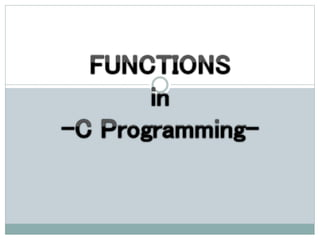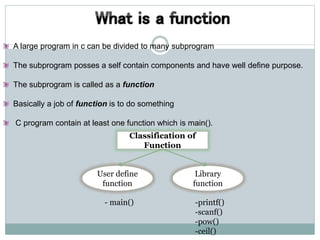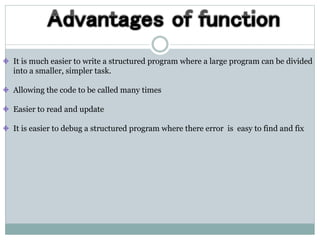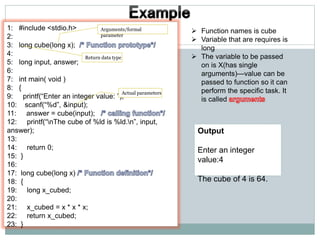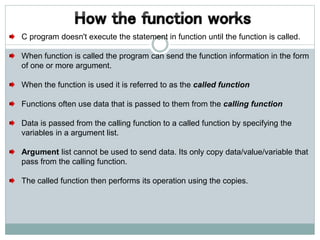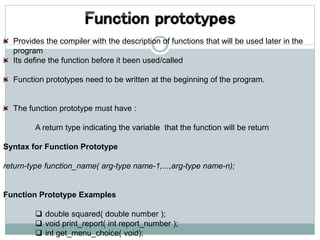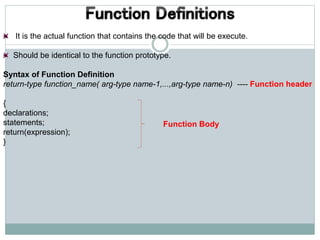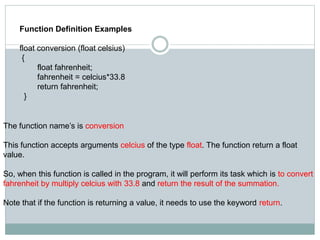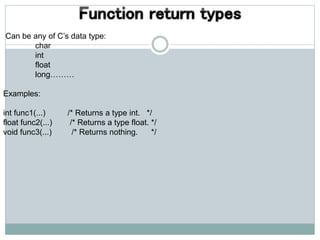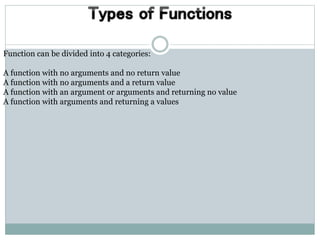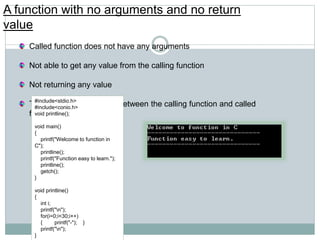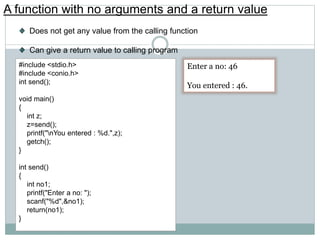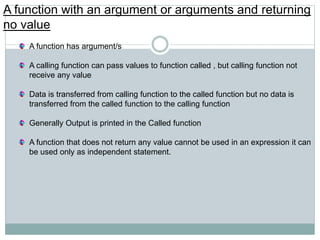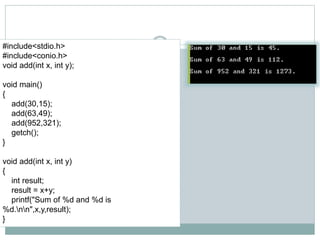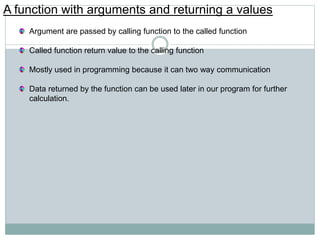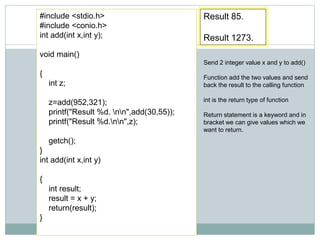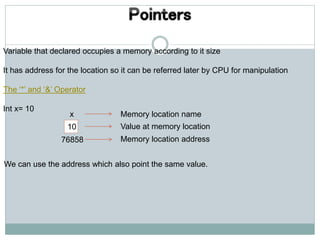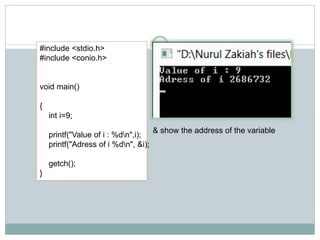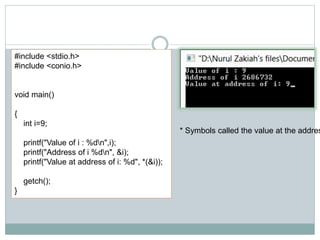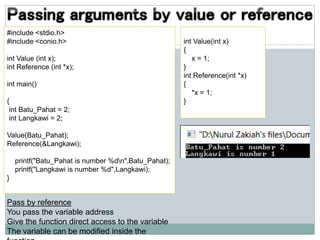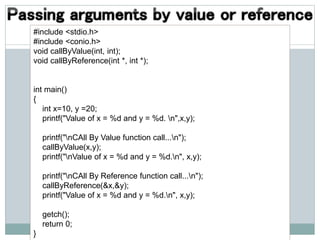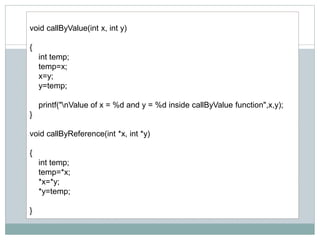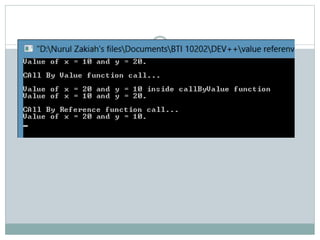1 von 23

### Function in c program

• 2. A large program in c can be divided to many subprogram The subprogram posses a self contain components and have well define purpose. The subprogram is called as a function Basically a job of function is to do something C program contain at least one function which is main(). Classification of Function Library function User define function - main() -printf() -scanf() -pow() -ceil()
• 3. It is much easier to write a structured program where a large program can be divided into a smaller, simpler task. Allowing the code to be called many times Easier to read and update It is easier to debug a structured program where there error is easy to find and fix
• 4. 1: #include <stdio.h> 2: 3: long cube(long x); 4: 5: long input, answer; 6: 7: int main( void ) 8: { 9: printf(“Enter an integer value: ”); 10: scanf(“%d”, &input); 11: answer = cube(input); 12: printf(“nThe cube of %ld is %ld.n”, input, answer); 13: 14: return 0; 15: } 16: 17: long cube(long x) 18: { 19: long x_cubed; 20: 21: x_cubed = x * x * x; 22: return x_cubed; 23: }  Function names is cube  Variable that are requires is long  The variable to be passed on is X(has single arguments)—value can be passed to function so it can perform the specific task. It is called Output Enter an integer value:4 The cube of 4 is 64. Return data type Arguments/formal parameter Actual parameters
• 5. C program doesn't execute the statement in function until the function is called. When function is called the program can send the function information in the form of one or more argument. When the function is used it is referred to as the called function Functions often use data that is passed to them from the calling function Data is passed from the calling function to a called function by specifying the variables in a argument list. Argument list cannot be used to send data. Its only copy data/value/variable that pass from the calling function. The called function then performs its operation using the copies.
• 6. Provides the compiler with the description of functions that will be used later in the program Its define the function before it been used/called Function prototypes need to be written at the beginning of the program. The function prototype must have : A return type indicating the variable that the function will be return Syntax for Function Prototype return-type function_name( arg-type name-1,...,arg-type name-n); Function Prototype Examples  double squared( double number );  void print_report( int report_number );  int get_menu_choice( void);
• 7. It is the actual function that contains the code that will be execute. Should be identical to the function prototype. Syntax of Function Definition return-type function_name( arg-type name-1,...,arg-type name-n) ---- Function header { declarations; statements; return(expression); } Function Body
• 8. Function Definition Examples float conversion (float celsius) { float fahrenheit; fahrenheit = celcius*33.8 return fahrenheit; } The function name’s is conversion This function accepts arguments celcius of the type float. The function return a float value. So, when this function is called in the program, it will perform its task which is to convert fahrenheit by multiply celcius with 33.8 and return the result of the summation. Note that if the function is returning a value, it needs to use the keyword return.
• 9. Can be any of C’s data type: char int float long……… Examples: int func1(...) /* Returns a type int. */ float func2(...) /* Returns a type float. */ void func3(...) /* Returns nothing. */
• 10. Function can be divided into 4 categories: A function with no arguments and no return value A function with no arguments and a return value A function with an argument or arguments and returning no value A function with arguments and returning a values
• 11. A function with no arguments and no return value Called function does not have any arguments Not able to get any value from the calling function Not returning any value There is no data transfer between the calling function and called function. #include<stdio.h> #include<conio.h> void printline(); void main() { printf("Welcome to function in C"); printline(); printf("Function easy to learn."); printline(); getch(); } void printline() { int i; printf("n"); for(i=0;i<30;i++) { printf("-"); } printf("n"); }
• 12. A function with no arguments and a return value Does not get any value from the calling function Can give a return value to calling program #include <stdio.h> #include <conio.h> int send(); void main() { int z; z=send(); printf("nYou entered : %d.",z); getch(); } int send() { int no1; printf("Enter a no: "); scanf("%d",&no1); return(no1); } Enter a no: 46 You entered : 46.
• 13. A function with an argument or arguments and returning no value A function has argument/s A calling function can pass values to function called , but calling function not receive any value Data is transferred from calling function to the called function but no data is transferred from the called function to the calling function Generally Output is printed in the Called function A function that does not return any value cannot be used in an expression it can be used only as independent statement.
• 14. #include<stdio.h> #include<conio.h> void add(int x, int y); void main() { add(30,15); add(63,49); add(952,321); getch(); } void add(int x, int y) { int result; result = x+y; printf("Sum of %d and %d is %d.nn",x,y,result); }
• 15. A function with arguments and returning a values Argument are passed by calling function to the called function Called function return value to the calling function Mostly used in programming because it can two way communication Data returned by the function can be used later in our program for further calculation.
• 16. Result 85. Result 1273. #include <stdio.h> #include <conio.h> int add(int x,int y); void main() { int z; z=add(952,321); printf("Result %d. nn",add(30,55)); printf("Result %d.nn",z); getch(); } int add(int x,int y) { int result; result = x + y; return(result); } Send 2 integer value x and y to add() Function add the two values and send back the result to the calling function int is the return type of function Return statement is a keyword and in bracket we can give values which we want to return.
• 17. Variable that declared occupies a memory according to it size It has address for the location so it can be referred later by CPU for manipulation The ‘*’ and ‘&’ Operator Int x= 10 x 10 76858 Memory location name Value at memory location Memory location address We can use the address which also point the same value.
• 18. #include <stdio.h> #include <conio.h> void main() { int i=9; printf("Value of i : %dn",i); printf("Adress of i %dn", &i); getch(); } & show the address of the variable
• 19. #include <stdio.h> #include <conio.h> void main() { int i=9; printf("Value of i : %dn",i); printf("Address of i %dn", &i); printf("Value at address of i: %d", *(&i)); getch(); } * Symbols called the value at the addres
• 20. #include <stdio.h> #include <conio.h> int Value (int x); int Reference (int *x); int main() { int Batu_Pahat = 2; int Langkawi = 2; Value(Batu_Pahat); Reference(&Langkawi); printf("Batu_Pahat is number %dn",Batu_Pahat); printf("Langkawi is number %d",Langkawi); } int Value(int x) { x = 1; } int Reference(int *x) { *x = 1; } Pass by reference You pass the variable address Give the function direct access to the variable The variable can be modified inside the
• 21. #include <stdio.h> #include <conio.h> void callByValue(int, int); void callByReference(int *, int *); int main() { int x=10, y =20; printf("Value of x = %d and y = %d. n",x,y); printf("nCAll By Value function call...n"); callByValue(x,y); printf("nValue of x = %d and y = %d.n", x,y); printf("nCAll By Reference function call...n"); callByReference(&x,&y); printf("Value of x = %d and y = %d.n", x,y); getch(); return 0; }
• 22. void callByValue(int x, int y) { int temp; temp=x; x=y; y=temp; printf("nValue of x = %d and y = %d inside callByValue function",x,y); } void callByReference(int *x, int *y) { int temp; temp=*x; *x=*y; *y=temp; }